Home > Uncategorized > From Acceleration to Displacement (part 2)

## From Acceleration to Displacement (part 2)

In a previous post I showed how to analytically double integrate (or rather repeat integrate) a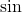wave to arrive at displacement or position. Here I’ll make it a little more generic, by integrating a sum ofwaves, each with its own amplitude and phase. This exercise is motivated by the fact that any arbitrarily complex waveform can be expressed as the sum of a number of sinusoids, so this will allow us to obtain the exact displacement when the acceleration is arbitrarily complex, as long as we’re able to determine (through FFT perhaps) the sinusoids that make up the acceleration.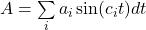First integration, to obtain velocity:

(1)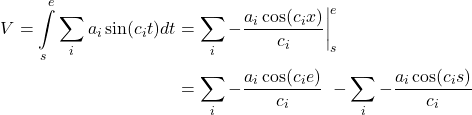Let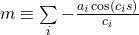and then do the second integration, to obtain displacement or position.

(2)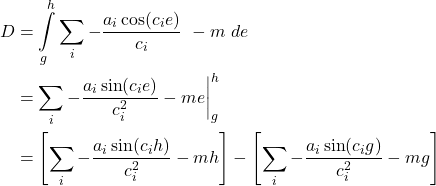Substitute everything back in (given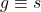and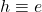), and simplify:

(3)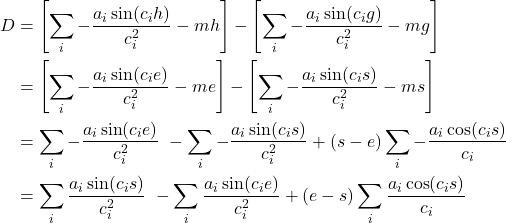Finally, to take the phase of the signal into account, we would start with: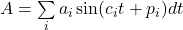And end with: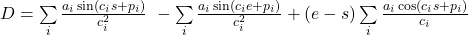Categories: Uncategorized Tags: